# Numbers up to 10-Digits

Numbers up to 10-Digits
Go back to  'Number Systems'

 1 Introduction to Numbers up to 10-Digits 2 The Big Idea: Numbers up to 10-Digits 3 How to Decompose 10-Digit Numbers? 4 How is it Important? 5 Comparison of the Indian System and the International System 6 Important Notes 7 Solved Examples on 10-Digit Numbers 8 Challenging Questions 9 Practice Questions 10 Maths Olympiad Sample Papers 11 Frequently Asked Questions (FAQs)

We at Cuemath believe that Math is a life skill. Our Math Experts focus on the “Why” behind the “What.” Students can explore from a huge range of interactive worksheets, visuals, simulations, practice tests, and more to understand a concept in depth.

Book a FREE trial class today! and experience Cuemath's LIVE Online Class with your child.

## Introduction to Numbers up to 10-Digits

There is a number so large that for you to count from 1 to that number, it would take years together.

If you constructed a cube with a volume equal to that number, the cube would have to be more than 80 feet tall.

The number we are talking about here is a Billion, and it is the smallest ten digit number, written as 1000000000

Just to give you a perspective about how large this number is, consider that your school is 3 miles from your house.

You must have read about the planets in school and must have heard of Pluto.

Well, the distance of the sun from Pluto is 3 billion miles.

You take half an hour to reach your school from your house.

So you would take a billion times more to reach the sun from Pluto.

## The Big Idea: Numbers up to 10-Digits

### A Simple Idea: The Importance of a Comma

As we said above, the smallest ten digit number is called a billion, and it is written as 1 followed by 9 zeros.

But just the different placement of the comma in two different number systems gives this number two different names.

The Indian System of numbers follows the 3:2:2 system of placing commas, so the smallest 10 digit number would be written as 1,00,00,00,000

Since the third comma after the 7th digit from right denotes the start of a crore, so this number would be called 100 crores (one Arab) in the Indian number system.

Let us now look at the 3:3 system of comma placement in the International number system. This number would be written as 1,000,000,000.

Since the third comma after the 9th digit from right denotes the start of a billion, so this number would be called 1 billion in the International number system.

## How to Decompose 10-Digit numbers?

Any ten digit number has place values up to one Arab (100 crores) or one billion.

These are the names of the place values (starting from the right) in a ten-digit number:

• Digit 1 – Units
• Digit 2 – Tens
• Digit 3 – Hundreds
• Digit 4 – Thousands
• Digit 5 – Ten Thousands
• Digit 6 –  Lakhs
• Digit 7 –  Ten Lakhs / Millions
• Digit 8 –  Crores / Ten Millions
• Digit 9 –  Ten Crores / Hundred Million
• Digit 10 – One Arab (Hundred Crores) / Billion

So let us take a random ten digit number - 5338652930, and see how it gets decomposed.

Here, PV stands for Place Value:

 Digit 1 PV = 0 x 1 = 0 Digit 2 PV = 3 x 10 = 30 Digit 3 PV = 9 x 100 = 900 Digit 4 PV = 2 x 1000 = 2000 Digit 5 PV = 5 x 10000 = 50000 Digit 6 PV = 6 x 100000 = 600000 Digit 7 PV = 8 x 1000000 = 8000000 Digit 8 PV = 3 x 10000000 = 30000000 Digit 9 PV = 3 x 100000000 = 300000000 Digit 10 PV = 5 x 1000000000 = 5000000000

## How is it Important?

### A 10-Digit Number We All Should Be Familiar With

The population of the world would soon be 8 billion.

To get an idea of how large that number is, digest the fact that even with so many companies making so many people so rich, at present there are only 140 people out of this 8 billion who are worth more than \$7 billion.

If this number was not big enough, just remember that the population of the world was 5 billion in 1987, and in the next twelve years, it became 6 billion, and in another 12 years, it crossed 7 billion.

The following facts will give you an idea of how large a number 7 billion is:

• If all the 7 billion people in the 2011 population had an average height of 5 feet (considering children as well), and were laid end-to-end, they would cover the distance to the moon 27 times and would go $${1 \over 14}$$ of the way to the sun.
• An ant weighs next to nothing, but if you were to weigh 7 billion ants together, they would together tip the scales at 23 tonnes!
• If someone walked along the earth's equator and took 7 billion steps, the diameter would be covered 106 times!!

That is how big the smallest 10-digit number is.

## Comparison of the Indian System and the International System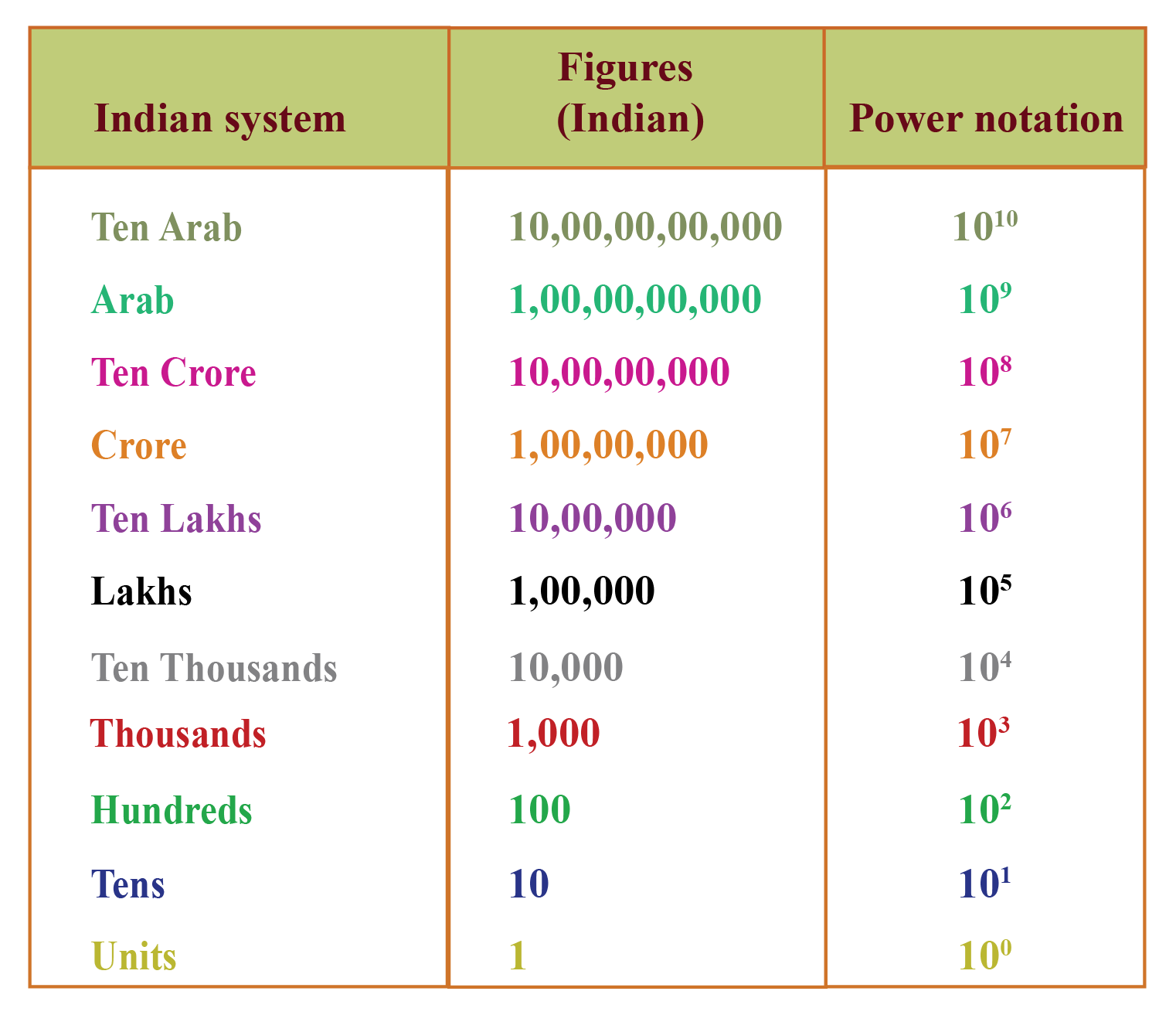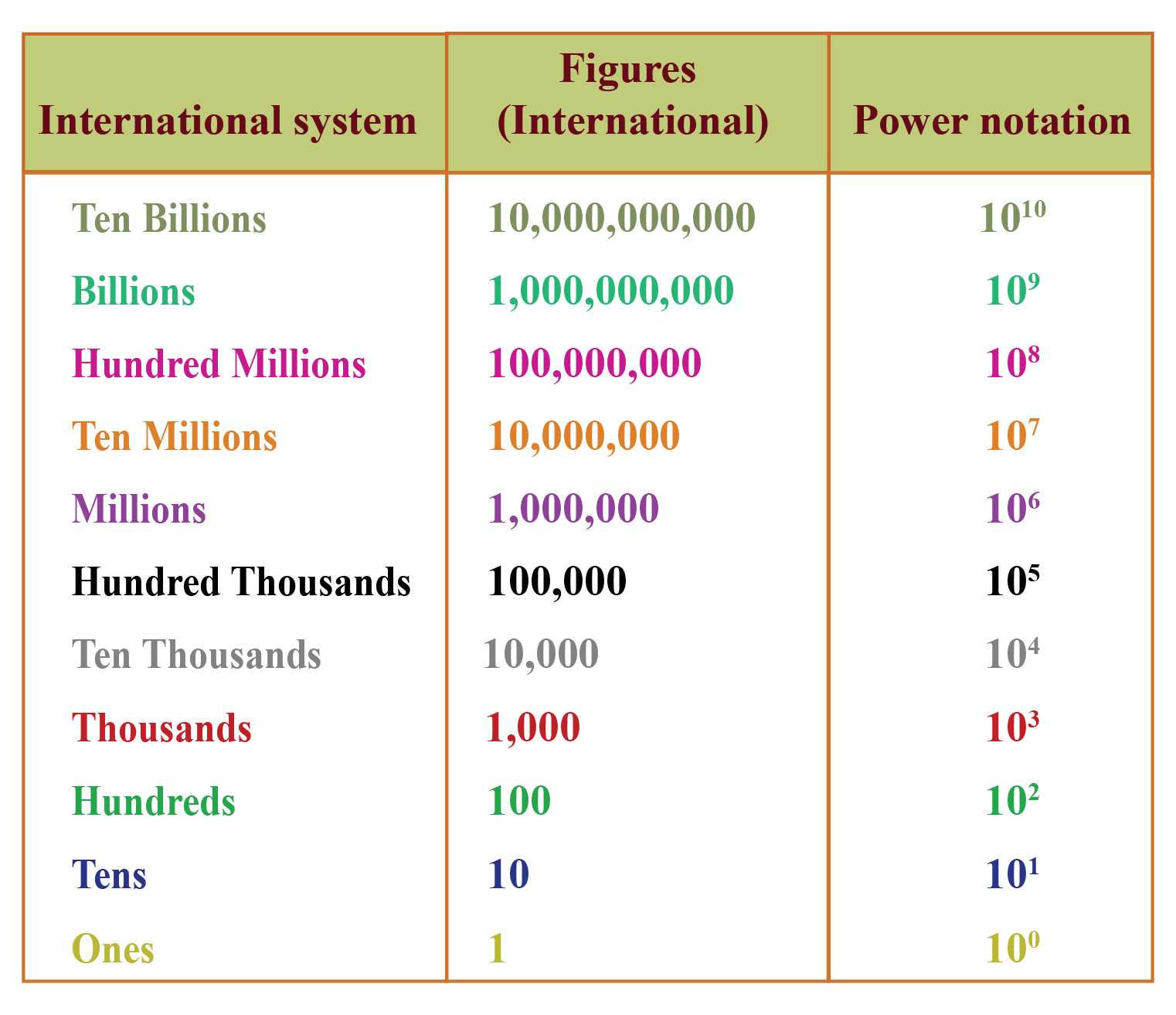Try the simulation shown below to see how the commas are placed in any number (Numbers up to 10-Digit) according to the Indian place value system and the International system.

Click on RESET, enter the number and click GO!

Help your child score higher with Cuemath’s proprietary FREE Diagnostic Test. Get access to detailed reports, customized learning plans, and a FREE counseling session. Attempt the test now.

## Solved Examples on 10-Digit Numbers

 Example 1

Write the following ten digit number in the expanded form (Indian place value system): 8,20,19,45,735

Solution:

8,20,19,45,735 can be expanded in three forms:

a.) 8,20,19,45,735 = 8 Arab + 2 ten crore + 0 crore + 1 ten lakh + 9 lakh + 4 ten thousands + 5 thousands + 7 hundreds + 3 tens + 5 ones

b.)

\begin{align} 8,20,19,45,735 &=\\ &(8\times 1000000000) +\\ &(2\times 100000000) +\\ &(0\times 10000000) +\\ &(1\times 1000000) +\\ &(9\times 100000) +\\&( 4\times 10000) + \\&(5\times 1000) + \\&(7\times 100) +\\ &(3 \times 10) + \\&(5\times 1) \end{align}

c.) 8,20,19,45,735 = 8,00,00,00,000 + 20,00,00,000 + 0 + 10,00,000 + 9,00,000 + 40,000 + 5,000 + 700 + 30 + 5

 Example 2

How is the number 6754349132 indicated with commas and read in the Indian place value system and the International Place value system?

Write the number names according to both the systems.

Solution:

The Indian system separates these numbers by adding commas in this way: 6,75,43,49,132

This is read as Six arab, seventy-five crore, forty-three lakh, forty-nine thousand, one hundred and thirty-two

The International system separates these numbers by adding commas in this way: 6,754,349,132

This is read as Six billion, seven hundred fifty-four million, three hundred forty-nine thousand, one hundred and thirty-two.

 Example 3

Find the two numbers with the help of the abacus.

Calculate their sum and place the commas according to the International place value system.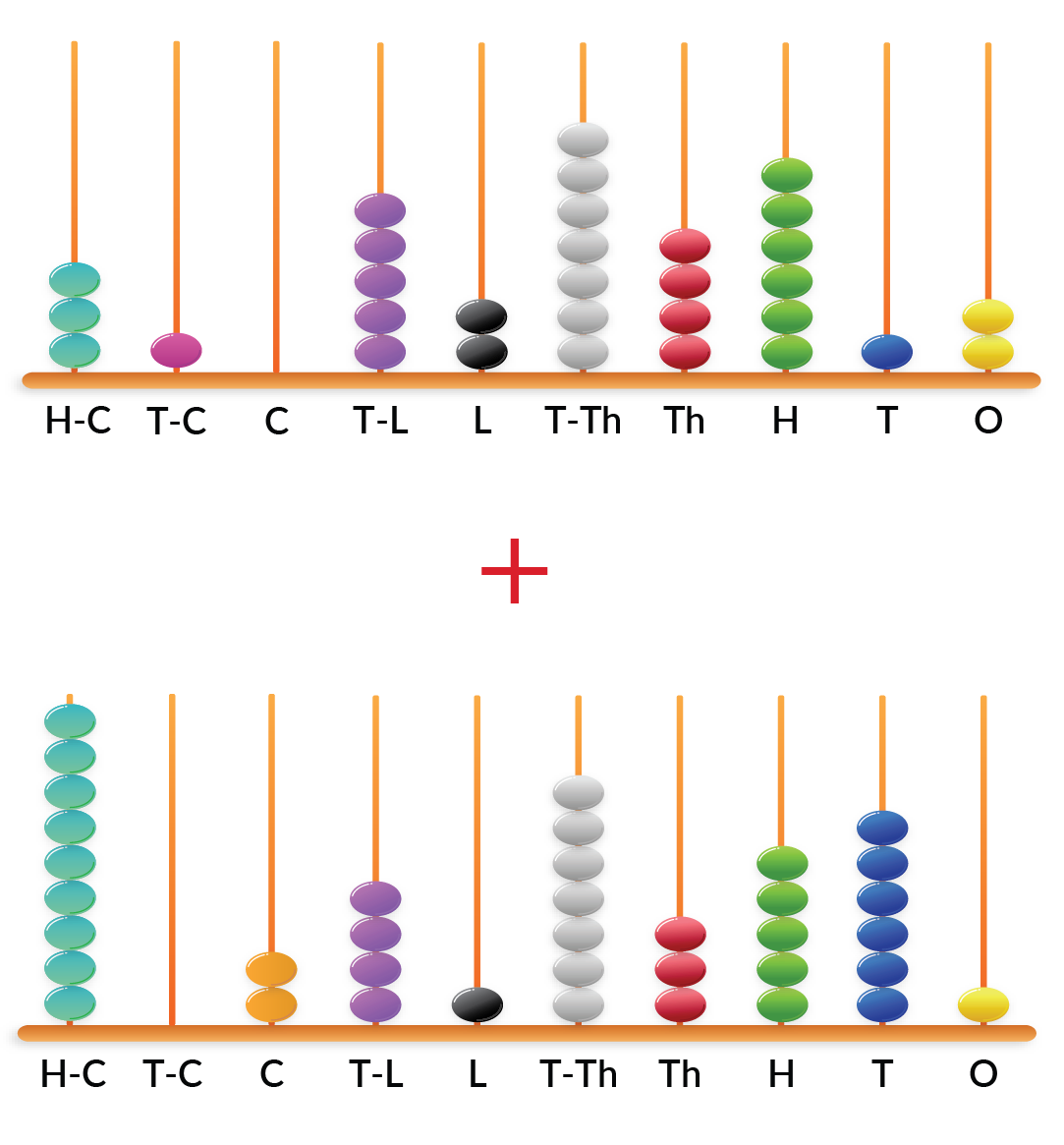Solution:

The number obtained from the first abacus: 3,105,274,612

The number obtained from the second abacus: 9,024,173,561

Their sum is: 3105274612 + 9024173561=12129448173

 $$\therefore$$ the sum is 12,129,448,173
 Example 4

Write the predecessor and successor of 3,45,75,42,009 along with their number names according to the Indian place value system.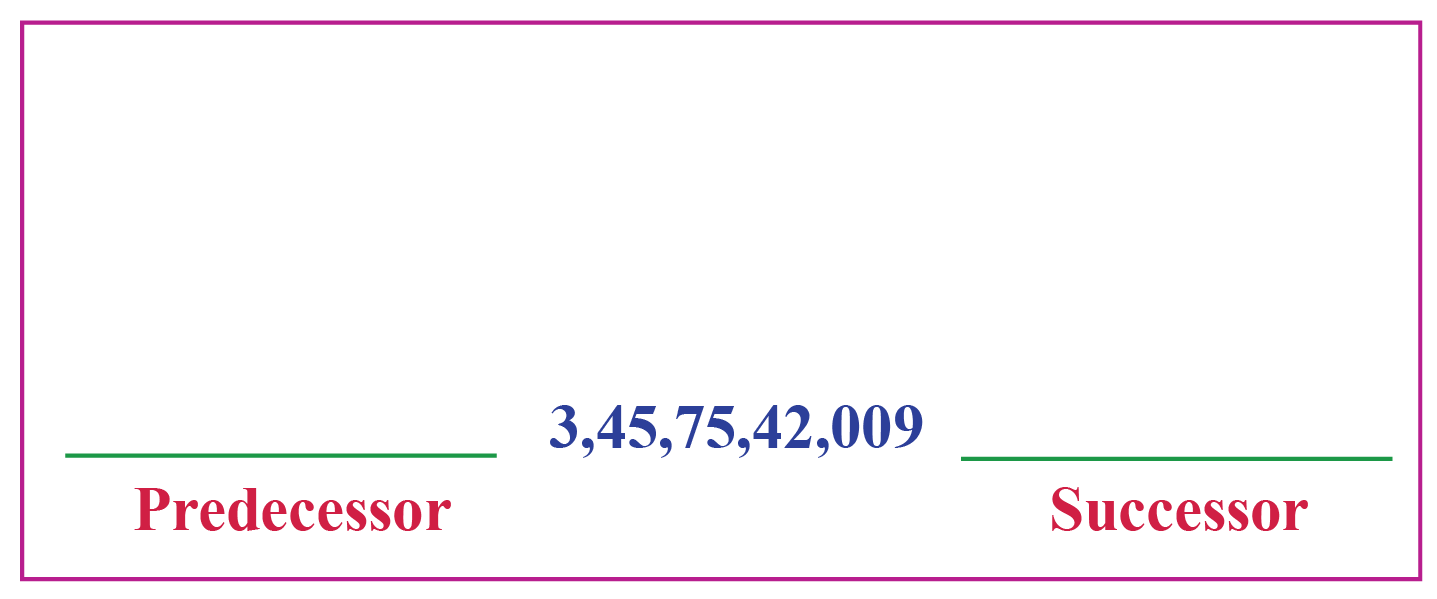Solution:

The predecessor of 3,45,75,42,009 is 3,45,75,42,008

Number name: Three arab, forty-five crore, seventy-five lakh, forty-two thousand and eight.

The successor of 3,45,75,42,009 is 3,45,75,42,010

Number name: Three arab, forty-five crore, seventy-five lakh, forty-two thousand and ten.

 Example 5

Find the difference between the largest 10-digit number and the smallest 8-digit number and write its predecessor as per the International place value system.

Solution:

The largest 10-digit number is 9,999,999,999

The smallest 8-digit number is 10,000,000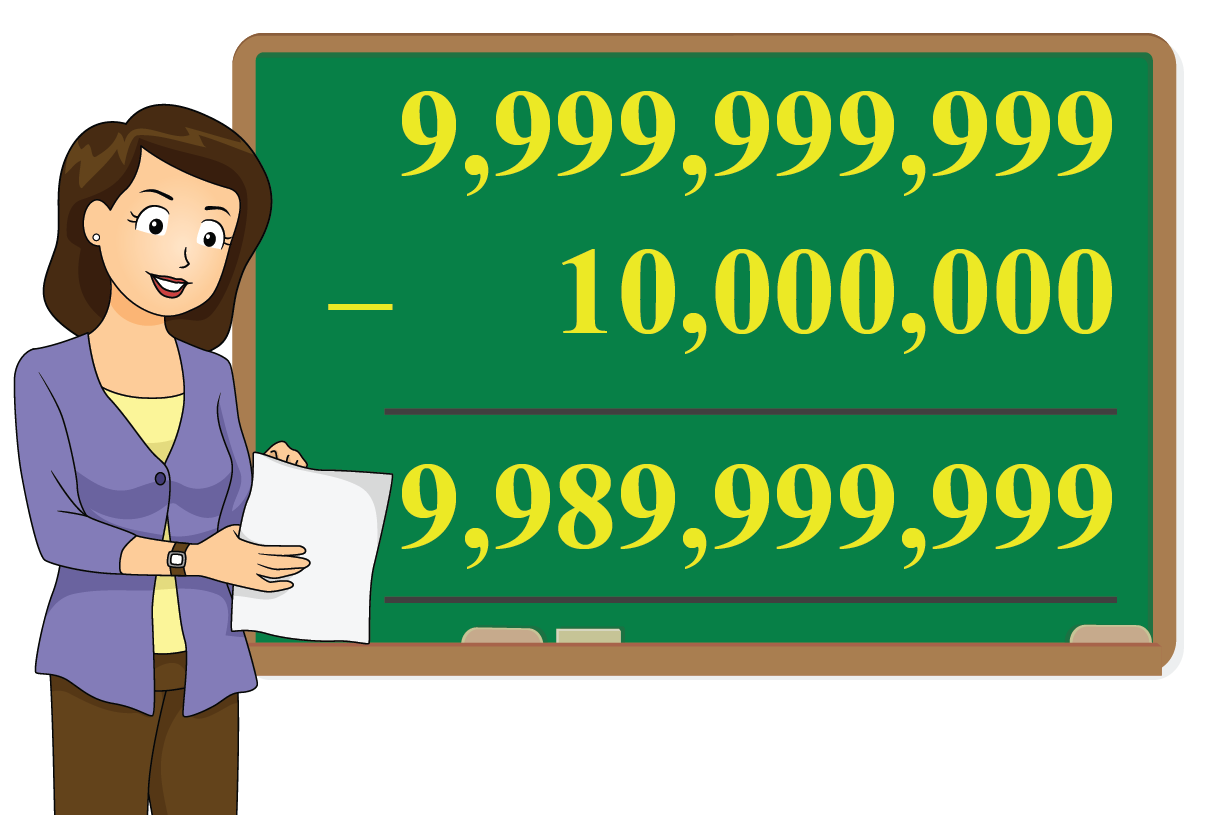Their difference is $$9,999,999,999-10,000,000 = \\ 9,989,999,999$$

The predecessor of 9,989,999,999 is 9,989,999,998

 $$\therefore$$ The predecessor is 9,989,999,998Challenging Questions

1. Find the 10-digit mystery number in which:

• 1 is in the Ten Thousands place
• 5 is in the Thousands place
• 4 is in the Tens place
• 8 is in the Ones place
• 7 is in the Hundreds place
• 6 is in the Billions place and the remaining place values are filled with 0

2. Arrange the following numbers in descending order: 6,767,776,667; 6,677,766,677; 6,776,667,676; 6,777,666,777

Have a doubt that you want to clear? Get it clarified with simple solutions on Numbers Up to 10 digits from our Math Experts at Cuemath’s LIVE, Personalised and Interactive Online Classes.

Make your kid a Math Expert, Book a FREE trial class today!

## Practice Questions

Here are a few activities for you to practice. Select/Type your answer and click the "Check Answer" button to see the result.

IMO (International Maths Olympiad) is a competitive exam in Mathematics conducted annually for school students. It encourages children to develop their math solving skills from a competition perspective.

## 1. Is a 10-digit number a billion?

Yes, a 10-digit number is read as a billion.

For example, 2,000,000,000 is read as Two billion.

## 2. How many digits are there in a billion?

There are 10 digits in a billion.

## 3. What is an 11-digit number?

An 11-digit number is a number which has 11 digits, where the first digit should be 1 or greater than 1 and the rest of the digits can be any number between 0-9

As per the International place value system, it starts from ten billion (10,000,000,000) and goes up to Ninety-nine billion, nine hundred ninety-nine million, nine hundred ninety-nine thousand, nine hundred ninety-nine (99,999,999,999).

## 4. How many zeros are there in 1 billion?

There are nine zeros in 1 billion (1,000,000,000).

## 5. One billion is equal to how many crores?

One billion is written as 1,000,000,000 as per the International place value system and is equal to Hundred Crores or One Arab (1,00,00,00,000) as per the Indian place value system.

## 6. How many commas are there in a billion?

The word "Billion" is used in the International place value system.

There are three commas in a billion (1,000,000,000).

## 7. What number is 1000000000?

This number is read as One billion according to the International place value system and read as One Arab (Hundred crores) as per the Indian place value system.

Introducing Numbers up to 10
Kg | Worksheet 1
Introducing Numbers up to 10
Kg | Worksheet 2

More Important Topics
Numbers
Algebra
Geometry
Measurement
Money
Data
Trigonometry
Calculus